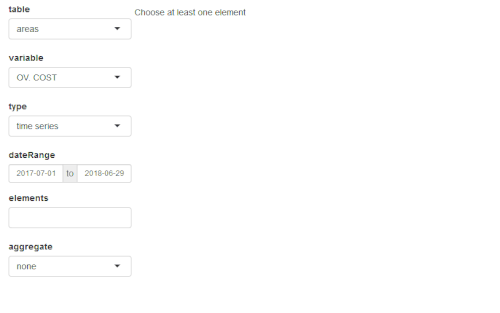# Quick presentation of antaresViz

#### 2018-10-11This package works along with RTE’s adequacy software ANTARES : https://antares.rte-france.com/

`antaresViz` is a package which proposes relevant graphs and maps to vizualize the results of an ANTARES study, with numerous settings and possible customizations.

## Where everything starts

``library(antaresViz)``

The data from an ANTARES study can be imported and easily manipulated using the antaresRead package.

The examples presented below have been build on a fictionnal study whose output have been loaded with antaresRead. They contains different types of elements :

• outputs with an annual and an hourly time step,
• synthetic outputs (averaged on several Monte-Carlo years) and detailled outputs (given year by year),
• outputs for areas, links, clusters and districts.
``load("data_for_antaresViz_vignette_extralight.Rdata")``

The aim of this vignette is to give a quick overview of the possibilities offered by the package antaresViz. The data with which the following graphs have been plotted are fictionnal.

## The simple power of the `plot` function

The `plot()` function, used with an antaresDataList object (i.e. an object returned by the `antaresRead::readAntares()` function) offers different possible vizualisations.

Moreover, this function is really easy to use. It opens a shiny interface which let the user decide :

• Which type of element he wants to study : areas, links or disctricts.
• Which variable he wants to analyze.
• With which type of graph : barplot, time series, probability density function, heatmap, etc.
• For which area(s), link(s), or district(s).
• And during which time period.
``plot(data_hourly_synthesis)``Note that all the interface manipulation can also be set directly in the arguments of the `plot` function. Some examples of the multiple graphs that can be returned by this function are given below.

###### Barplot of Loss of Load Duration (LOLD) for several districts of the study
``plot(data_annual_synthesis, table = "districts" ,variable = "LOLD", type = "barplot", elements = c("00_a", "00_b", "00_c", "00_d", "00_e", "00_f", "00_g", "00_h", "00_i"), interactive = FALSE, width = "100%", height = 400)``
###### Time series of the load in an area with average value and 95% confidence interval.
``plot(data_hourly_allmc, table = "areas" ,variable = "LOAD", type = "ts", elements = "23_b", confInt = 0.95, dateRange = c("2018-01-08", "2018-01-14"), width = "100%", height = 400, interactive = FALSE)``
###### Probability density function of the wind power generation in two areas
``plot(data_hourly_synthesis, table = "areas" ,variable = "WIND", type = "density", elements = c("01_a", "02_a"), interactive = FALSE, width = "100%", height = 400)``
###### Heatmap of the congestion probability of one interconnection
``plot(data_hourly_synthesis_1year, table = "links", variable = "CONG. PROB +", type = "heatmap", elements = "25_c - 26_d", interactive = FALSE, width = "100%", height = 400, main = "Congestion probability")``

Note that the `plot()` function also contains a `compare` argument which allows comparisons between :

• different variables
• different studies

## When the production meets the demand

The `prodStack` function builds a graph which contains the time series of demand in one area (or the sum of the demand of a set of areas) along with the generation of this area (or set of areas), divided between the different fuel types (e.g. nuclear, gas, wind, etc).

``prodStack(data_hourly_synthesis)``

Once again, this function is easy to use and opens a shiny interface which let the user manipulate the selected areas and time range. Some settings can also be passed to the function through its (optionnal) arguments.

``prodStack(data_hourly_synthesis, stack = "eco2mix", areas = "37_h", dateRange = c("2018-01-08", "2018-01-21"), main = "Production stack", unit = "GWh",  interactive = FALSE, width = "100%", height = 500)``

The graphical template used by default is the one of the RTE’s application eco2mix. This template can though be redefined completely by the user with the function `setProdStackAlias()`.

The `exchangesStack()` function proposes similar graphs with a superposition of all the imports and exports of an area.

``exchangesStack(data_hourly_synthesis, area = "37_h", dateRange = c("2018-01-08", "2018-01-21"), main = "Import/Export of area 37_h", unit = "GWh",  interactive = FALSE, width = "100%", height = 500)``

## Everything looks better on a map

Last but not least, `antaresViz` proposes several function to vizualise the results of a study on a map.

To do so, the first function to use is `mapLayout()`. This function launches an interactive application that let the user place areas of the ANTARES study on a map.

``````antares_layout <- antaresRead::readLayout(opts = antaresRead::setSimulationPath(study_path))
map_layout <- mapLayout(layout = antares_layout)``````